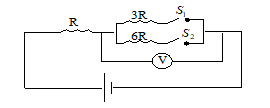voltmeter
Question

# In the circuit shown in the figure, reading of voltmeter is ${\mathrm{V}}_{1}$  when only ${\mathrm{S}}_{1}$ is closed, reading of voltmeter is ${\mathrm{V}}_{2}$ when only ${\mathrm{S}}_{2}$  is closed and reading of voltmeter is ${\mathrm{V}}_{3}$ when both  are closed. Then,Moderate
Solution

## In series P.D is directly proportional to ‘R’ When ${\mathrm{S}}_{1}$  is closed  When ${\mathrm{S}}_{2}$  is closed  When both are closed  ${V}_{2}>{V}_{1}>{V}_{3}$

Get Instant Solutions